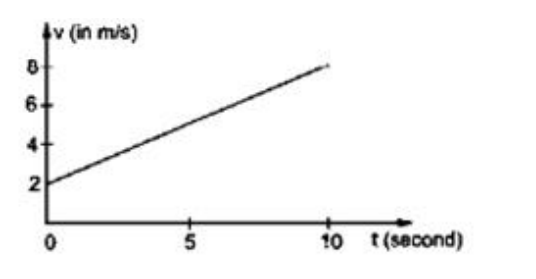# Solve the following :

Question:

The given figure shows the graph of velocity versus time for a particle going along the X-axis. Find

(a) The acceleration

(b) The distance travelled in 0 to 10 s and

(c) The displacement in 0 to $10 \mathrm{~s}$

Solution:(a) Acceleration=slope of $v-t$ graph

$=\frac{(8-2)}{10}=0.6 \mathrm{~m} / \mathrm{s}^{2}$

(b) Distance travelled = Area under v-t graph

$=\frac{1}{2}(2+8) 10$

$=50 \mathrm{~m}$

(c) Displacement $=50 \mathrm{~m}$